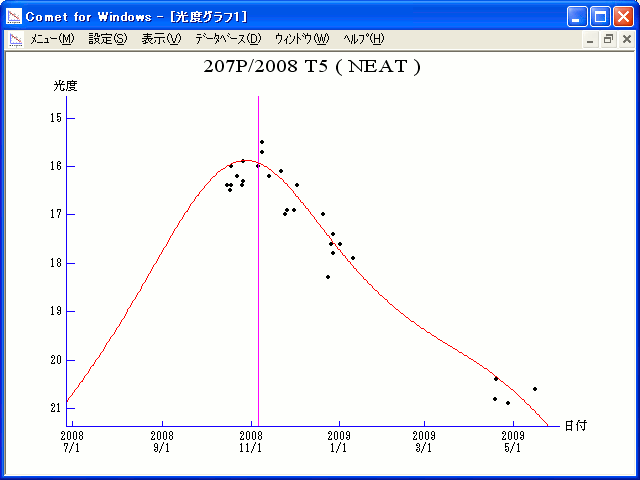# \$B%K!<%HWB@1(B

207P/NEAT (2008)###\$B%W%m%U%#!<%k(B

 \$BId9f(B 207P/2008 T5 \$B8!=PF|(B 2008\$BG/(B10\$B7n(B15\$BF|(B \$B8!=P8wEY(B 16.4\$BEy(B \$B8!=P \$BLgED7r0l(B (\$B:k6L8)>eHx;T(B)

###\$B###\$B50F;MWAG(B

```                    Epoch = 2008 Oct. 21.0 TT
T = 2008 Nov.  6.2551 TT         Peri. = 271.1732
e = 0.757141                     Node  = 200.6744  2000.0
q = 0.944153 AU                  Incl. =  10.1500
a =  3.887655 AU    n = 0.1285797    P =   7.665 years
```

###\$B@1?^(B###\$B8wEYJQ2=(B

```        m1 = 16.0 + 5 log\$B&\$(B + 10.0 log r
```##### \$B50F;MWAG\$O!"(BIAUC 8996\$B\$K7G:\\$5\$l\$?\$b\$N\$G\$9!#(B \$B@1?^\$O%9%F%i%J%S%2!<%?(B Ver.8 (\$B%"%9%H%m%"!<%D(B) \$B\$G:n@.\$7\$?\$b\$N\$G\$9!#(B \$B8wEY%0%i%U\$O(BComet for Windows\$B\$G:n@.\$7\$?\$b\$N\$G\$9!#(B